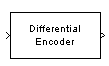# Differential Encoder

Encode binary signal using differential coding

## Library

Source Coding

•## Description

The Differential Encoder block encodes the binary input signal within a channel. The output is the logical difference between the current input element and the previous output element. More specifically, the input and output are related by

d(i0) = m(i0) XOR Initial condition parameter value

d(ik) = d(ik-1) XOR m(ik)

where

• m is the input message.

• d is the differentially encoded output.

• ik is the kth element.

• XOR is the logical exclusive-or operator.

This block accepts a scalar or column vector input signal and treats columns as channels.

## Parameters

Initial conditions

The logical exclusive-or of this value with the initial input value forms the initial output value.

## Supported Data Type

PortSupported Data Types
In
• Double-precision floating point

• Single-precision floating point

• Boolean

• Integer

• Fixed-point

Out
• Double-precision floating point

• Single-precision floating point

• Boolean

• Integer

• Fixed-Point

## References

 Couch, Leon W., II, Digital and Analog Communication Systems, Sixth edition, Upper Saddle River, N. J., Prentice Hall, 2001.

## Pair Block

Differential Decoder

## Version History

Introduced before R2006a